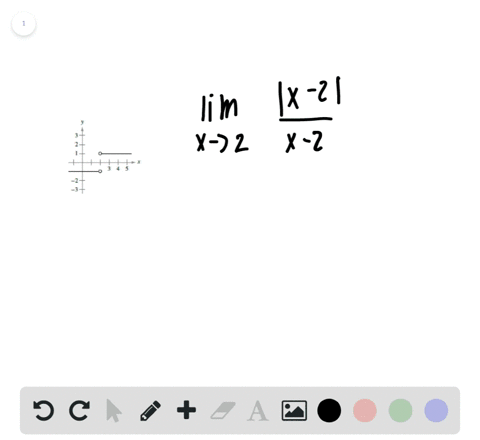Enroll in one of our FREE online STEM bootcamps. Join today and start acing your classes!View Bootcamps00:41
Problem 24

# In Exercises 21-28, use the graph to find the limit (if it exists). If the limit does not exist, explain why.$$\lim _{x \rightarrow 1} f(x)$$

## Discussion

You must be signed in to discuss.

## Video Transcript

and this problem were asked to find the limit as X approaches one of f of X. Where is defined to be this piece wise function. So we want to graphically compute this limit. So to our left, we have the graph effect of vexes sketch of that graph, Mom. So we'll use this to find the limited sex purchase one. So if we look at the graph and we look at the behavior uplevel vex from the left one, we see that half of it is approaching positive for And when we look at the right, the behavior from the right hand side of X equals one. You see that F of X is also approaching for so we say the left hand women is for and the right hand limit is fourth. Since these two limits agree and they have a finite values, we can say the limit has X approaches. One is for and that's your answer. Um, so just note, we'll limit as X perches. One is not equal to half of one. We see from the scrap here. That and one it's equal too, too, actually, because it's represented by the filled in doc assistance after one is equal. 22 We say that filament just not green with the function value in this problem.